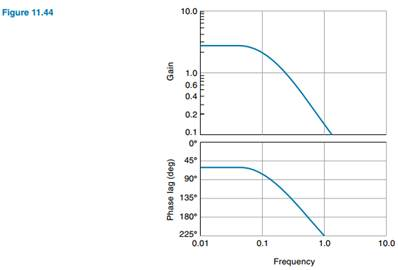### Create an Account

Home / Questions / The Bode plot for a control system is shown in Figure 1144 Is this system stable Find the ...

# The Bode plot for a control system is shown in Figure 1144 Is this system stable Find the gain margin and phase margin

The Bode plot for a control system is shown in Figure 11.44. Is this system stable? Find the gain margin and phase margin.Jul 15 2020 View more View LessSubscribe To Get Solution